Updating search results...

# 7 Results

View
Selected filters: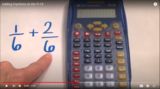Unrestricted Use
CC BY
Rating
0.0 stars

This brief video shows students how to enter a fraction into the TI-15 calculator and to add fractions. Students should access to a TI-15 calculator while viewing, This video may be embedded within Google Classroom.

Subject:
Mathematics
Material Type:
Activity/Lab
Diagram/Illustration
Interactive
Teaching/Learning Strategy
Author:
Dawne Coker
06/28/2020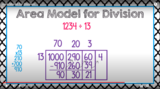Unrestricted Use
CC BY
Rating
0.0 stars

This is one possible method for using the area model for division.

With this division strategy, students divide by breaking the dividend into its expanded form. Then, students use familiar multiplication facts to divide. It is suggested that this would be the first of three strategies for division (preceding partial quotients and the standard algorithm.

Subject:
Mathematics
Material Type:
Activity/Lab
Diagram/Illustration
Interactive
Teaching/Learning Strategy
Author:
Dawne Coker
06/28/2020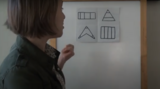Unrestricted Use
Public Domain
Rating
0.0 stars

This lesson is Day 1 in a series of 12 lessons around fraction equivalences and comparisons.

This video lesson encourages students to reason about and visualize fractions. The lesson focuses on the ideas that fractions are fair shares and that fractions can be compared. This lesson assumes that students have some initial experiences with fractions.

Subject:
Mathematics
Material Type:
Interactive
Lesson
Presentation
Simulation
Author:
Dawne Coker
06/25/2020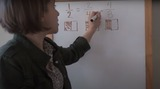Unrestricted Use
Public Domain
Rating
0.0 stars

This lesson is Day 2 in a series of 12 lessons around fraction equivalences and comparisons.

This video lesson uses paper folding to introduce the concept of equivalent fractions. To complete this lesson, make sure you have a square piece of paper (any size) and a crayon

Subject:
Mathematics
Material Type:
Activity/Lab
Interactive
Lesson
Simulation
Author:
Dawne Coker
06/25/2020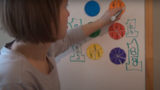Unrestricted Use
CC BY
Rating
0.0 stars

This lesson is Day 2 in a series of 12 lessons around fraction equivalences and comparisons.

This video lesson introduces the idea that fractions can be used to represent 1 whole. To engage in this lesson, students may use virtual circle models from Toy Theater (https://toytheater.com/fraction-circles/)

Subject:
Mathematics
Material Type:
Activity/Lab
Interactive
Lesson
Simulation
Author:
Dawne Coker
06/25/2020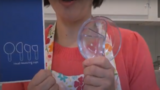Unrestricted Use
CC BY
Rating
0.0 stars

This lesson is Day 4 in a series of 12 lessons around fraction equivalences and comparisons.

This video lesson looks at how we use fractions in the kitchen. Students are challenged to use circle models to find equivalent fractions for a recipe. Students may use virtual circe models from the Toy Theater (https://toytheater.com/fraction-circles/).

Subject:
Mathematics
Material Type:
Activity/Lab
Interactive
Lesson
Simulation
Author:
Dawne Coker
06/25/2020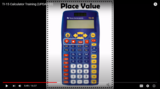Unrestricted Use
CC BY
Rating
0.0 stars

This video demonstrates the functions of the TI-15 calculator. It may be used as a professional development for teachers, or for training students. It is not recommended that the video is shown to students in its entirety. If you scroll down to the comments section under the video, time markers for each function are provided. Click on the time markers to go directly to that specific function.

Subject:
Mathematics
Material Type:
Activity/Lab
Diagram/Illustration
Interactive
Teaching/Learning Strategy
Author:
Dawne Coker